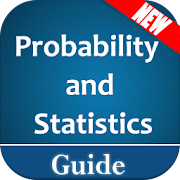# Probability and Statistics - Lumos Educational App Store4.41
Price -fREE
\$0

#### DESCRIPTION:

Probability and Statistics or also called Statistics and Probability are two related but separate academic disciplines. Statistical analysis often uses probability distributions, and the two topics are often studied together. ... Moreover, many topics in statistics are independent of probability theory. Introduction to Probability and Statistics with terms & definitions of essential topics. It will help students who want to learn Probability and Statics. Here is the full topics list you will able to learn from this app: Statistics: Introduction Basic Definitions Statistics: Introduction

#### OVERVIEW:

Probability and Statistics is a free educational mobile app By .It helps students in grades HS practice the following standards HSS.ID.A.2.

This page not only allows students and teachers download Probability and Statistics but also find engaging Sample Questions, Videos, Pins, Worksheets, Books related to the following topics.

1. HSS.ID.A.2 : Use statistics appropriate to the shape of the data distribution to compare center (median, mean) and spread (interquartile range, standard deviation) of two or more different data sets..

HS

#### STANDARDS:

HSS.ID.A.2

Developer:

Software Version: 2.1.2

Category:

### RELATED APPSEdSearch WebSearch